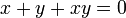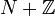## Definition

Suppose$N$ is an radical ring, i.e., an associative ring where, for every$x \in N$, there exists$y \in N$ such that$x + y + xy = 0$. Note that$N$ cannot be a unital ring, because it's not possible to find a$y$ that works for$-1$.

First, make$N$ a semigroup with the operation$x * y := x + y + xy$ (this is the multiplicative formal group law, but unlike other formal group laws, it works even without commutativity assumptions). The adjoint group of$N$ is the subgroup of this semigroup comprising the elements with two-sided inverses. The identity element for the adjoint group is$0 \in N$.

### 1 plus notation

For convenience, and to avoid confusing the elements of$N$ with the same elements viewed as elements of the adjoint group, we denote the adjoint group element for$x \in N$ as the formal expression$1 + x$. The advantage of this is that the group multiplication now arises formally from the multiplication in$N$ and distributivity, i.e.:$(1 + x)(1 + y) = 1 + x + y + xy = 1 + (x * y)$

### Interpretation inside unitization

The adjoint group can be thought of concretely as the coset$1 + N$ for$N$ of$1$ in the unitization$N + \mathbb{Z}$. Alternatively, if$N$ is an algebra over a commutative unital ring$R$, the adjoint group can be thought of as the coset$1 + N$ for$N$ of$1$ in the unitization$1 + N$.